Updated by Julianna Biggs on Nov 09, 2018
REPORTJulianna Biggs
Owner
10 items   1 followers   0 votes   6 views

Fractions with Addition and Subtraction

Here are some different sites you can let your students use when teaching about fractions with addition and subtraction.

1

Website at https://www.mathsisfun.com/fractions.html

Description - This website allows students to connect fractions to something they know in real life- pizza. It, also, teaches students about the terms and goes over how to add fractions with like and unlike denominators.
Rationale - I chose this resource because it has a lot of models with fractions - and addition of fractions - using pizzas. It also bolds the key terms so students will know which words are important to know.

2

Speedway Fractions | MathPlayground.com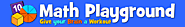Description: This game is about adding and subtracting fractions with like denominators and being able to quickly reduce fractions.
Rational: This game will be fun for the students and make the learning process slightly more fun. It is not too difficult for students who are just learning this topic, but it is difficult enough to challenge the students. At the end, you can see your speed and challenge yourself to beat it.

3

Adding Fractions | 5th grade | Math | Khan AcademyDescription: This video talks about how to add two fractions with unlike denominators using a visual representation, a box divided.
Rational: I chose to use this video because some of my students will be visual learners and they may be able to better understand after watching this video.

4

Subtracting Fractions | 5th grade | Math | Khan AcademyDescription: This video talks about how to subtract two fractions with unlike denominators using visual representations of boxes that have been divided.
Rational: I chose this video because some students are visual learners and will better understand the concept of subtracting unlike fractions after viewing this video.

5

Adding & Subtracting Fractions | Sciencing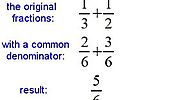Description: I will be using the picture posted above in my Hyperdocs.
Rational: The picture above shows students the process of adding fractions. This will be beneficial because students can use this whenever needed.

6

Subtracting Fractions - EnchantedLearning.com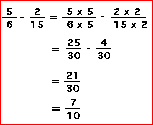Desciption: I will use the above picture in my HyperDocs to show my students the right way to subtract and the method to get to the solution.
Rational: The picture above will help show the students the correct way to get to the solution. This will help the students who are more visual.

7

Math Games: Fruit Splat Fractions Subtraction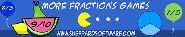Description: This is a subtracting fractions game with different levels and styles.
Rational: This has 3 different levels, each separated into A and B. The students can work there way up through the varying levels, or I can have my higher functioning students play one level and my lower functioning students will play another.

8

Math Games: Fruit Splat Fractions AdditionDescription: This is an adding fractions game with different levels and styles.
Rational: This has 3 different levels, each separated into A and B. The students can work there way up through the varying levels, or I can have my higher functioning students play one level and my lower functioning students will play another.

9

Adding and Subtracting Fractions - Practice Flashcards | QuizletDescription: This weebly allows students to practice their addition and subtraction of fractions.
Rationale: The weebly is just the addition and subtraction of fractions. They can study it with flashcards or with the games.

10

Adding And Subtracting Fractions Quiz - ProProfs Quiz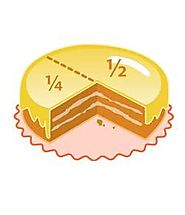Description: The HyperDocs will end with the students taking this quiz and then sending a nimbus screenshot of their final results.
Rationale: This will help me realize how the students learned and let me know if I need to go any more in depth with the topic.